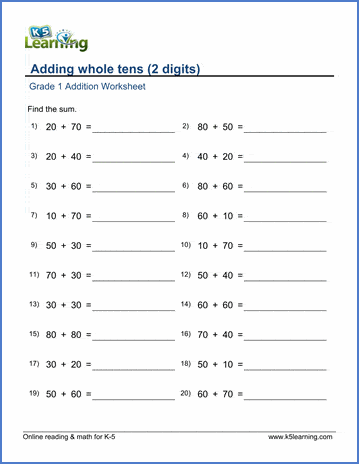Topics include:

• Adding numbers up to 10 or 20 by counting objects
• Practice addition facts (sums to 5, 10 or 20)
• Making 10 from two single digit numbers
• Adding 10 to a single digit number
• Missing addend questions (sums to 10)

• Adding numbers up to 10 or 30 by counting pictured objects
• Addition sentences & number lines
• Addition of single digit numbers (sums to 10, 20 ...)
• Number bonds (sums 8 to 10)
• Adding doubles and near doubles
• Missing addends (sums to 10, 20)
• Adding 3 numbers within 20
• Completing the next ten
• Adding 2-digit and 1 digit numbers mentally (no carrying)
• Adding numbers in columns (1-2 digit numbers)
• Mixed addition and subtraction word problems

• Adding by completing whole tens
• Adding 1 and 2 digit numbers (no regrouping)
• Adding 1 and 2 digit numbers (with regrouping)
• Adding 3 or 4 numbers
• Completing the next ten
• Adding whole tens & whole hundreds
• Adding whole tens to 2-digit or 3-digit numbers
• Adding 1 and 3 digit numbers
• Adding two to four 2-digit numbers in columns
• Adding 3 digit numbers in columns
• Mixed addition and subtraction word problems

• Adding 1, 2 and 3 digit numbers
• Adding whole tens to two digit numbers
• Completing 100, whole hundreds and whole thousands
• Adding 2-4 digit numbers in columns

• Mental addition of 2 digit numbers (with / without regrouping)
• Completing whole hundreds and whole thousands
• Adding 3-6 digit numbers in columns
• Mixed addition and subtraction word problems
• Mixed 4 operations word problems

• Adding 4-6 digit numbers in columns
• Adding very large numbers in columns
• Mixed 4 operations word problems

## Related topics

Subtraction worksheets

Multiplication worksheets